“众志已成城，风卷红旗如画。”要说，中国汽车工业史最让人魂牵梦萦的第一国车，一定非红旗莫属。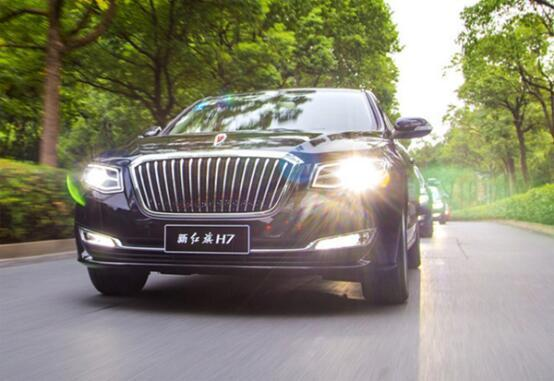在国人眼里，红旗早已超越了一个汽车品牌的含义，而是代表着国家的形象，承载着国人的重托和期望。红旗，这两个字乃是几代人的梦想和坚持，这一抹红色也代表着行动和担当，承载着意志和理想。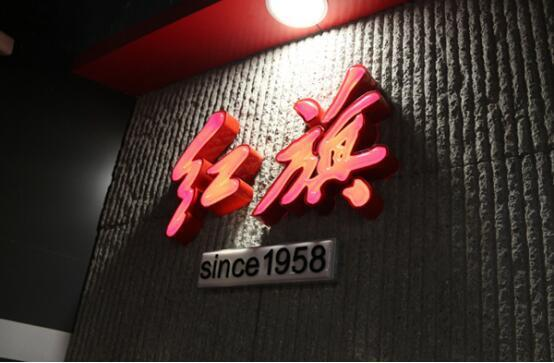当年历翻篇至2018年，承载了几代国人汽车梦的红旗，会有何翻天覆地的变化吗？答案是肯定的。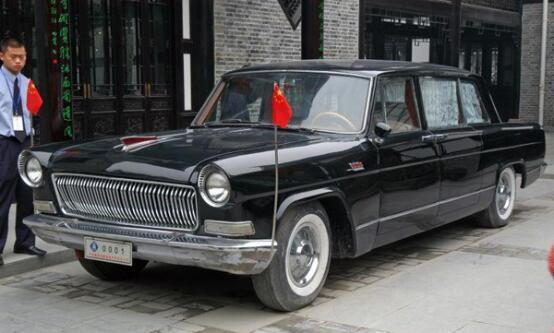“新红旗 让梦想成真”——中国一汽红旗品牌战略发布会将于1月8日在北京人民大会堂举行。届时，中国一汽将发布红旗品牌的未来发展战略，其中包括红旗汽车未来的造型理念、红旗新的产品序列等重磅信息。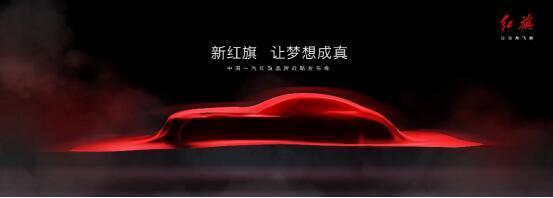从发布会主题不难看出，这次红旗铿锵有力地许下了“让梦想成真”的承诺。

60载理想，终迎复兴

诞生于1958年的红旗轿车，在中国家喻户晓，它在今年也将迎来自己的60岁生日。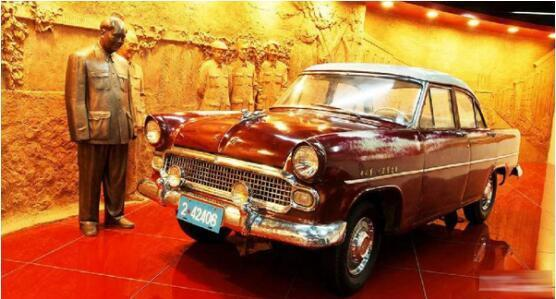1958年5月，中国一汽生产出了第一台高档轿车“东风”CA71，在当年9月更名为“红旗”； 1959年5月正式挂标上路，代号CA72；从此红旗轿车成为了中国汽车工业的代名词，并于1960年被写入了《世界汽车年鉴》。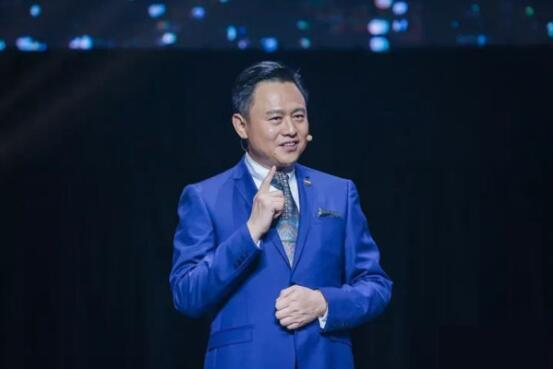从2008年开始，一汽正式启动“红旗复兴”项目，坚持正向开发、自主创新，自主研发成功H、L两大系列新一代红旗轿车。

不过，如今红旗品牌荣耀复兴的时刻，真的已经渐行渐近了。

特别是新任中国第一汽车集团董事长、党委书记徐留平到任后，一汽红旗连续在品牌、产品、渠道营销体系建设以及多元合作等方面发生着翻天覆地的变化。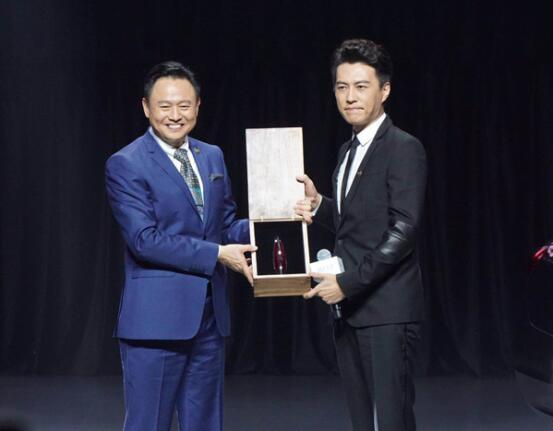9月9日，中国一汽15万人召开“我心中的红旗”大讨论总结大会，为红旗振兴建言献策。9月18日，一汽集团宣布组织构架大调整，总部直接运营红旗品牌。9月21日，在新红旗H7上市仪式现场，徐留平满怀深情地说，“红旗的情怀、红旗的梦想在我心中，在一汽人心中、在无数中国人心中，一直在飘扬。”

他继而斩钉截铁地表态：将集一汽集团之力，把红旗品牌真正打造成中国第一豪华品牌。

伴随着大刀阔斧的革新，一汽振兴红旗终于迎来了绝佳的时间窗口，红旗新战略的前奏也被接连释放。

大不一样，豪华新生

在中国一汽红旗品牌战略发布会现场，红旗诸多“求新求变”的元素，都将让人惊爆眼球。

在人民大会堂举办，与会人数超过670人，这意味着将是一场充满家国情怀的、名副其实的“国字号”盛典。

从此前网间曝出的海报看，不仅郎平、靳东、黄豆豆、李云迪等名人要来，还有来自互联网行业、创投行业等跨大咖。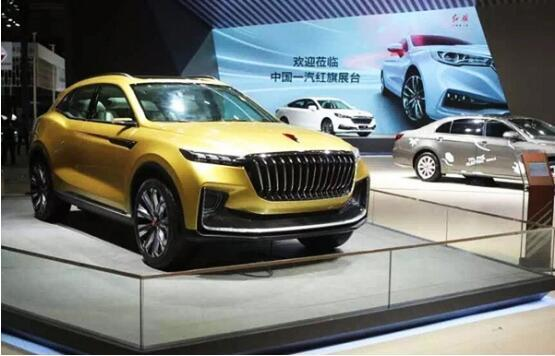众多明星名人现身，显然将为红旗品牌的荣耀复兴打call；跨行业大咖云集，则透露出红旗品牌未来将整合全球优势资源，在前瞻共性技术创新、汽车全价值链运营、联合出海“走出去”、新商业模式等领域共拓创新。

在品牌层面，红旗品牌的全新理念即将发布，但不难推测的是，中国式、高尚、精致将成为核心关键词，而其用户也将瞄准 “中国式新精英专业情怀人士”。为此，红旗作出了一次前所未有的尝试——邀请靳东出任红旗品牌代言人。靳东拥有长腿、帅脸、低音炮三大醒目标签，再加上他正派的说话行事作风，颇有一股“老干部”之风，与红旗的品牌形象很是契合，也很快拉近了红旗与年轻群体的距离。

据此前公布信息显示，到2020年红旗将推出8款新品，覆盖从B级到E级轿车、SUV以及高端商务礼宾车等各个细分市场。在三大平台和十余款车型的产品研发矩阵下，红旗品牌的高竞争力产品都将陆续投放市场。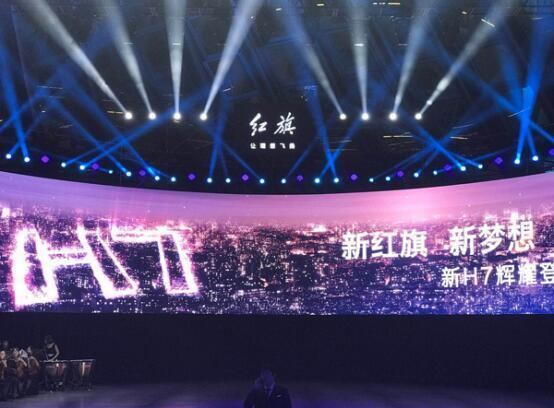在品牌方面，在融合经典与现代元素的全新品牌形象下，一方面，红旗检阅车、定制车的超豪华格调将得以传承和加强；另一方面，更具时代感的年轻化要素则将谱写出红旗全新的品牌变革新篇章。

在技术方面，红旗拥有自主开发的V12、V8、V6和四缸增压系列发动机，以及全新开发的底盘系统、电子电气、网络平台、车身与内外饰等，工艺技术也具有独到优势，达到国际先进水平。此外，在PHEV、智能网联、安全技术等方面的积累，都将为红旗品牌未来的新豪华，注入底气十足的技术基因。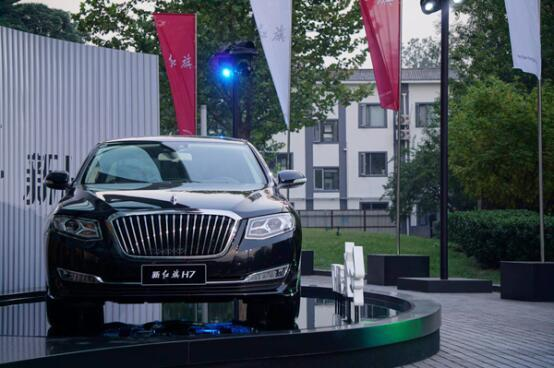可以说，在徐留平铿锵有力的振兴红旗的口号下， 红旗的豪华印记将不再只是停留在表面上，在全新设计理念和全体系支撑之下，它的复兴大业将一步一个脚印地走下去。

随着1月8日的一汽红旗品牌战略全盘托出，复兴红旗品牌，把其打造成驰名中外的中国式高档车品牌，将不再是一句口号，而是造梦者更脚踏实地的付诸行动。

中国已经成为全球豪华汽车增长最快的市场，虽说国外豪华汽车品牌悉数进入，并占据了绝对垄断地位，但红旗品牌要想打破垄断，脱颖而出，也完全存在机会。当然啦，需要调动一切积极因素，调动一切资源，上下同心，艰苦努力、持之以恒地去拼搏、去创新，才能取得最后的成功。（车透社原创文章，主编罗裕）
##### 相关资讯

|分享

•已阅！握爪
•我手滑为你点赞
•128个赞！
•不明觉厉
•阅后既醉
•有钱！任性
•照片太美，我不敢看
•干货！杠杠的
•高大上
•膜拜中！
•请接受我的膝盖
•猫哥/猫妹么么哒
•神吐槽
•我只笑笑不说话
•我想静静
•窒息
##### 资讯相关车系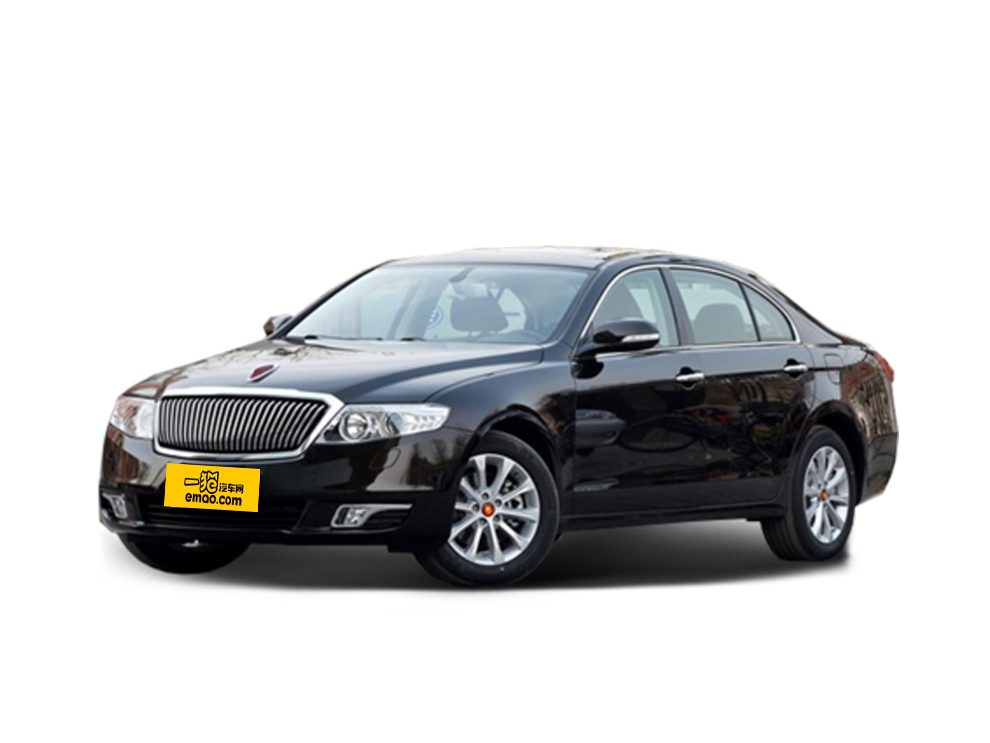##### 热度排行• 阿斯顿·马丁
• 奥迪
• 阿尔法罗密欧
• ALPINA

• 巴博斯
• 宝骏
• 宝马
• 保时捷
• 北汽制造
• 奔驰
• 奔腾
• 本田
• 比亚迪
• 标致
• 别克
• 宾利
• 布加迪
• 北汽威旺
• 北京
• 北汽绅宝
• 北汽幻速
• 北汽新能源
• 宝沃
• 比速汽车
• 北汽道达

• 昌河
• 长安
• 长城
• 长安商用
• 成功汽车
• 长江EV
• 长安轻车型

• 大众
• 道奇
• 东风
• 东风风神
• 东风小康
• 东南
• DS
• 东风风行
• 东风风度
• 东风风光

• 法拉利
• 菲亚特
• 丰田
• 福迪
• 福特
• 福田汽车
• 福汽启腾
• 风诺

• GMC
• 广汽传祺
• 广汽吉奥
• 观致

• 哈飞
• 海格
• 海马
• 华泰
• 黄海
• 恒天
• 红旗
• 哈弗
• 华颂
• 华凯
• 华泰新能源
• 汉腾汽车

• Jeep
• 江淮
• 江铃
• 捷豹
• 金杯
• 九龙
• 吉利汽车
• 金旅
• 金龙
• 江铃集团轻汽
• 江铃集团新能源
• 君马汽车
• 捷途

• 开瑞
• 凯迪拉克
• 科尼赛克
• 克莱斯勒
• KTM
• 卡威
• 凯翼
• 康迪
• 康迪电动汽车集团

• 猎豹汽车
• 兰博基尼
• 劳斯莱斯
• 雷克萨斯
• 雷诺
• 理念
• 力帆
• 莲花汽车
• 林肯
• 铃木
• 陆风
• 路虎
• 路特斯
• 领志
• 领克
• 零跑汽车

• MG
• MINI
• 马自达
• 玛莎拉蒂
• 迈凯伦
• 摩根

• 纳智捷

• 讴歌
• 欧宝
• 欧朗
• 欧拉
• 欧尚汽车

• 帕加尼

• 奇瑞
• 启辰
• 起亚
• 前途
• 庆铃汽车

• 日产
• 荣威
• 瑞麒汽车
• 如虎
• 瑞驰

• 上汽大通
• smart
• 三菱
• 双环
• 双龙
• 斯巴鲁
• 斯柯达
• 萨博
• 思铭
• 赛麟
• SWM斯威汽车

• TESLA
• 泰卡特
• 腾势

• 威麟
• 威兹曼
• 沃尔沃
• 五菱汽车
• 五十铃
• 潍柴英致
• WEY
• 蔚来
• 威马汽车

• 现代
• 雪佛兰
• 雪铁龙
• 西雅特
• 新特汽车
• 小鹏汽车
• 新宝骏

• 野马汽车
• 一汽
• 依维柯
• 英菲尼迪
• 永源
• 驭胜

• 中华
• 中兴
• 众泰
• 知豆
• 之诺
• 正道汽车
• A
• B
• C
• D
• E
• F
• G
• H
• I
• J
• K
• L
• M
• N
• O
• P
• Q
• R
• S
• T
• U
• V
• W
• X
• Y
• Z

• 阿斯顿·马丁
• 奥迪
• 阿尔法罗密欧
• ALPINA

• 巴博斯
• 宝骏
• 宝马
• 保时捷
• 北汽制造
• 奔驰
• 奔腾
• 本田
• 比亚迪
• 标致
• 别克
• 宾利
• 布加迪
• 北汽威旺
• 北京
• 北汽绅宝
• 北汽幻速
• 北汽新能源
• 宝沃
• 比速汽车
• 北汽道达

• 昌河
• 长安
• 长城
• 长安商用
• 成功汽车
• 长江EV
• 长安轻车型

• 大众
• 道奇
• 东风
• 东风风神
• 东风小康
• 东南
• DS
• 东风风行
• 东风风度
• 东风风光

• 法拉利
• 菲亚特
• 丰田
• 福迪
• 福特
• 福田汽车
• 福汽启腾
• 风诺

• GMC
• 广汽传祺
• 广汽吉奥
• 观致

• 哈飞
• 海格
• 海马
• 华泰
• 黄海
• 恒天
• 红旗
• 哈弗
• 华颂
• 华凯
• 华泰新能源
• 汉腾汽车

• Jeep
• 江淮
• 江铃
• 捷豹
• 金杯
• 九龙
• 吉利汽车
• 金旅
• 金龙
• 江铃集团轻汽
• 江铃集团新能源
• 君马汽车
• 捷途

• 开瑞
• 凯迪拉克
• 科尼赛克
• 克莱斯勒
• KTM
• 卡威
• 凯翼
• 康迪
• 康迪电动汽车集团

• 猎豹汽车
• 兰博基尼
• 劳斯莱斯
• 雷克萨斯
• 雷诺
• 理念
• 力帆
• 莲花汽车
• 林肯
• 铃木
• 陆风
• 路虎
• 路特斯
• 领志
• 领克
• 零跑汽车

• MG
• MINI
• 马自达
• 玛莎拉蒂
• 迈凯伦
• 摩根

• 纳智捷

• 讴歌
• 欧宝
• 欧朗
• 欧拉
• 欧尚汽车

• 帕加尼

• 奇瑞
• 启辰
• 起亚
• 前途
• 庆铃汽车

• 日产
• 荣威
• 瑞麒汽车
• 如虎
• 瑞驰

• 上汽大通
• smart
• 三菱
• 双环
• 双龙
• 斯巴鲁
• 斯柯达
• 萨博
• 思铭
• 赛麟
• SWM斯威汽车

• TESLA
• 泰卡特
• 腾势

• 威麟
• 威兹曼
• 沃尔沃
• 五菱汽车
• 五十铃
• 潍柴英致
• WEY
• 蔚来
• 威马汽车

• 现代
• 雪佛兰
• 雪铁龙
• 西雅特
• 新特汽车
• 小鹏汽车
• 新宝骏

• 野马汽车
• 一汽
• 依维柯
• 英菲尼迪
• 永源
• 驭胜

• 中华
• 中兴
• 众泰
• 知豆
• 之诺
• 正道汽车
• A
• B
• C
• D
• E
• F
• G
• H
• I
• J
• K
• L
• M
• N
• O
• P
• Q
• R
• S
• T
• U
• V
• W
• X
• Y
• Z

• 阿斯顿·马丁
• 奥迪
• 阿尔法罗密欧
• ALPINA

• 巴博斯
• 宝骏
• 宝马
• 保时捷
• 北汽制造
• 奔驰
• 奔腾
• 本田
• 比亚迪
• 标致
• 别克
• 宾利
• 布加迪
• 北汽威旺
• 北京
• 北汽绅宝
• 北汽幻速
• 北汽新能源
• 宝沃
• 比速汽车
• 北汽道达

• 昌河
• 长安
• 长城
• 长安商用
• 成功汽车
• 长江EV
• 长安轻车型

• 大众
• 道奇
• 东风
• 东风风神
• 东风小康
• 东南
• DS
• 东风风行
• 东风风度
• 东风风光

• 法拉利
• 菲亚特
• 丰田
• 福迪
• 福特
• 福田汽车
• 福汽启腾
• 风诺

• GMC
• 广汽传祺
• 广汽吉奥
• 观致

• 哈飞
• 海格
• 海马
• 华泰
• 黄海
• 恒天
• 红旗
• 哈弗
• 华颂
• 华凯
• 华泰新能源
• 汉腾汽车

• Jeep
• 江淮
• 江铃
• 捷豹
• 金杯
• 九龙
• 吉利汽车
• 金旅
• 金龙
• 江铃集团轻汽
• 江铃集团新能源
• 君马汽车
• 捷途

• 开瑞
• 凯迪拉克
• 科尼赛克
• 克莱斯勒
• KTM
• 卡威
• 凯翼
• 康迪
• 康迪电动汽车集团

• 猎豹汽车
• 兰博基尼
• 劳斯莱斯
• 雷克萨斯
• 雷诺
• 理念
• 力帆
• 莲花汽车
• 林肯
• 铃木
• 陆风
• 路虎
• 路特斯
• 领志
• 领克
• 零跑汽车

• MG
• MINI
• 马自达
• 玛莎拉蒂
• 迈凯伦
• 摩根

• 纳智捷

• 讴歌
• 欧宝
• 欧朗
• 欧拉
• 欧尚汽车

• 帕加尼

• 奇瑞
• 启辰
• 起亚
• 前途
• 庆铃汽车

• 日产
• 荣威
• 瑞麒汽车
• 如虎
• 瑞驰

• 上汽大通
• smart
• 三菱
• 双环
• 双龙
• 斯巴鲁
• 斯柯达
• 萨博
• 思铭
• 赛麟
• SWM斯威汽车

• TESLA
• 泰卡特
• 腾势

• 威麟
• 威兹曼
• 沃尔沃
• 五菱汽车
• 五十铃
• 潍柴英致
• WEY
• 蔚来
• 威马汽车

• 现代
• 雪佛兰
• 雪铁龙
• 西雅特
• 新特汽车
• 小鹏汽车
• 新宝骏

• 野马汽车
• 一汽
• 依维柯
• 英菲尼迪
• 永源
• 驭胜

• 中华
• 中兴
• 众泰
• 知豆
• 之诺
• 正道汽车
• A
• B
• C
• D
• E
• F
• G
• H
• I
• J
• K
• L
• M
• N
• O
• P
• Q
• R
• S
• T
• U
• V
• W
• X
• Y
• Z